### Artificial Neural Networks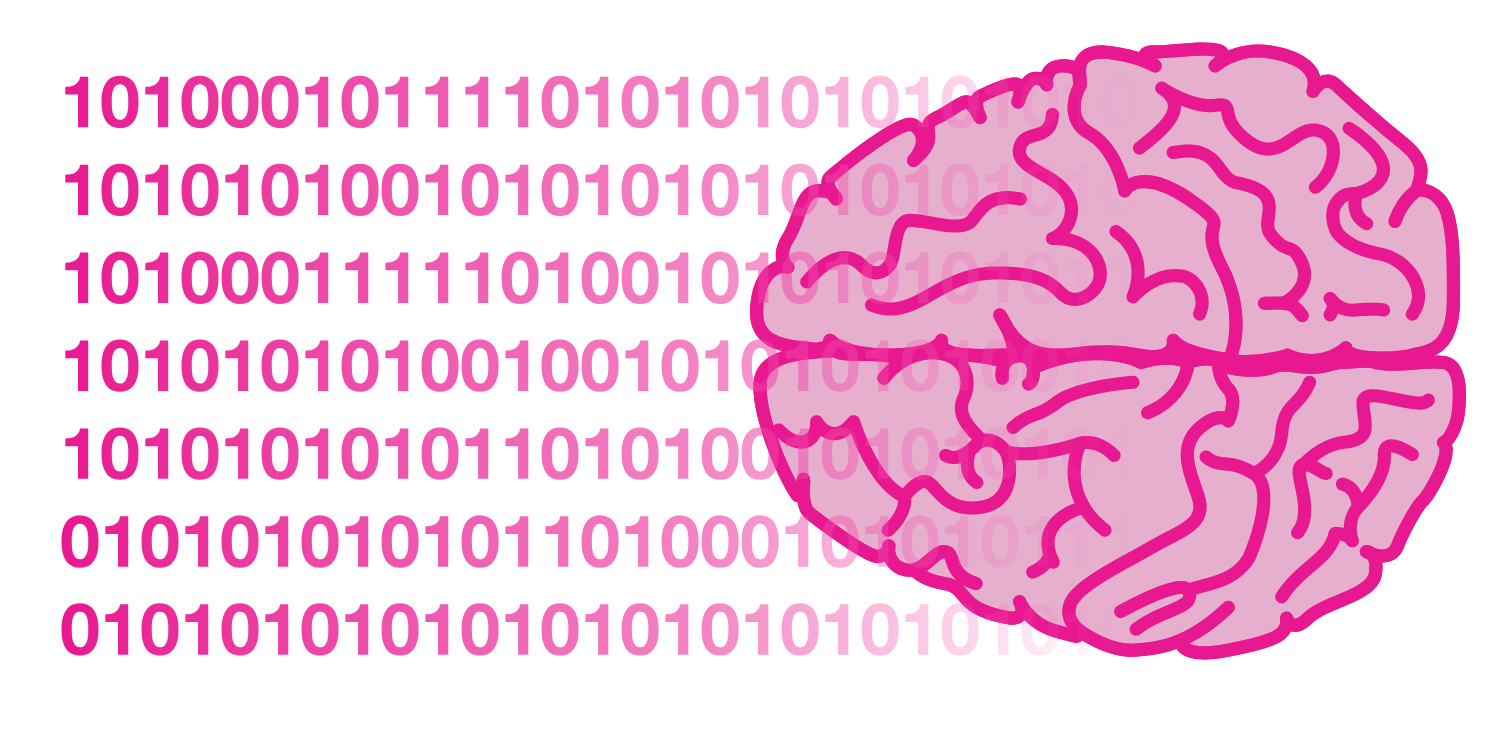When training a learning model, there are two main processes that can be used with respect to how the training data is handled: batch learning and online learning.

In batch learning, the model learns from batches of data — often from the entire training set at once. In online learning, the model learns from data processed sequentially over time as it becomes available.

Which type of learning does the human brain mainly use?

# Computationally Modeling The Brain

Which of the following is not an advantage of online learning over batch learning? Try to use your intuition.

# Computationally Modeling The Brain

For both practical and theoretical reasons, ANNs can actually use either (or both) of online learning and batch learning. Some of these tradeoffs will be explored later in this course.

Now that we’ve discussed how the brain (and ANNs) might import data, what does it actually do with this data to learn? This isn’t a neuroanatomy course, so straight to the point: neurons are the simplest units of computation in the human brain, and their interactions facilitate brain functions such as learning.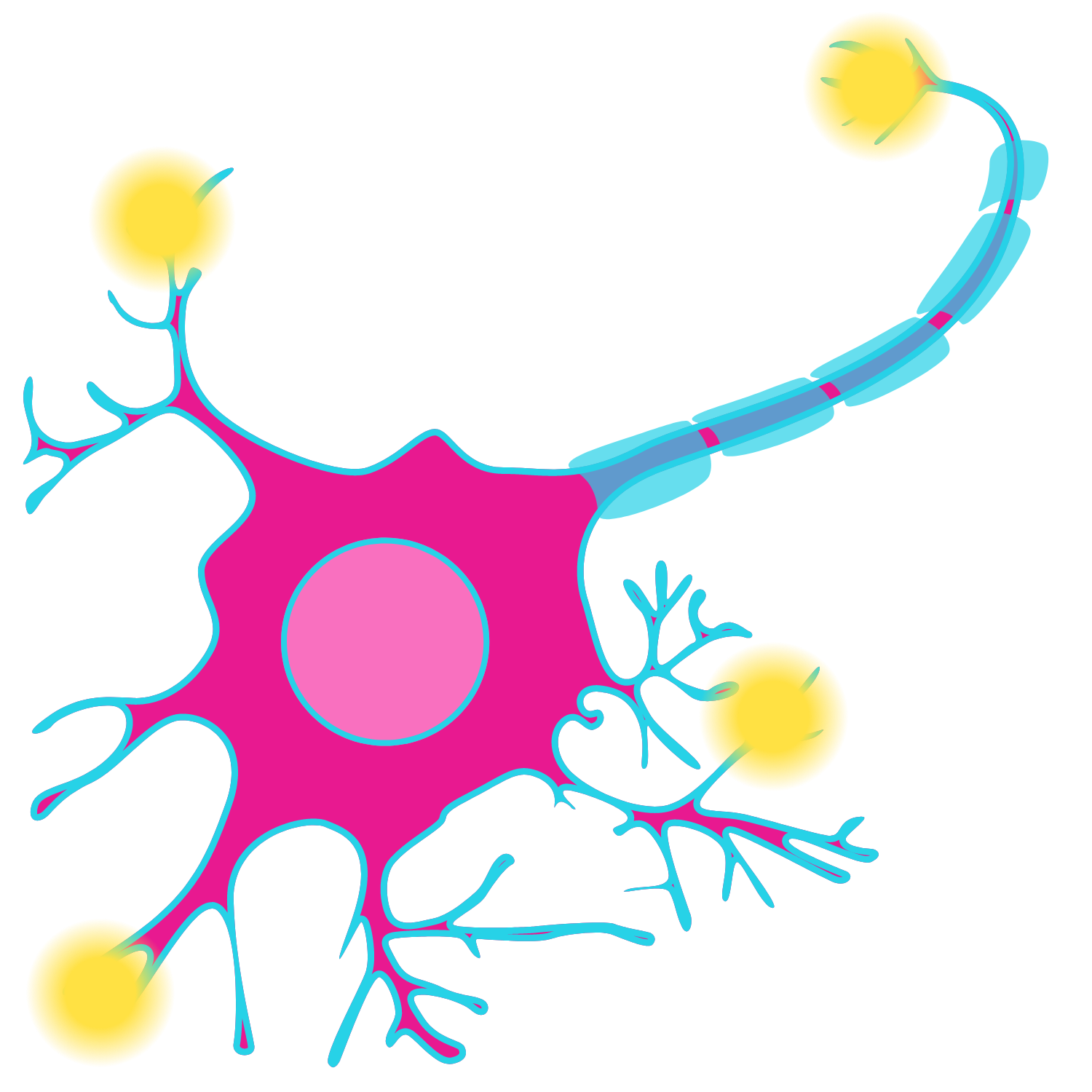Neurons each compute a simple function. While the actual dynamics of a neuron's computation are complex, a simplified view of them is that they integrate and fire. That is, a neuron performs a computation with its inputs and then fires if that computation passes a certain threshold.

# Computationally Modeling The Brain

The basic idea of the neuron model in ANNs is that the inputs to a neuron are combined (as a weighted sum) into a single value, $v.$ Then, an activation function, $H(v),$ is applied to determine whether or not the neuron fires.

For a physical neuron, the firing is “all-or-nothing”; that is, it fires or it doesn’t. Which activation function below would best model this?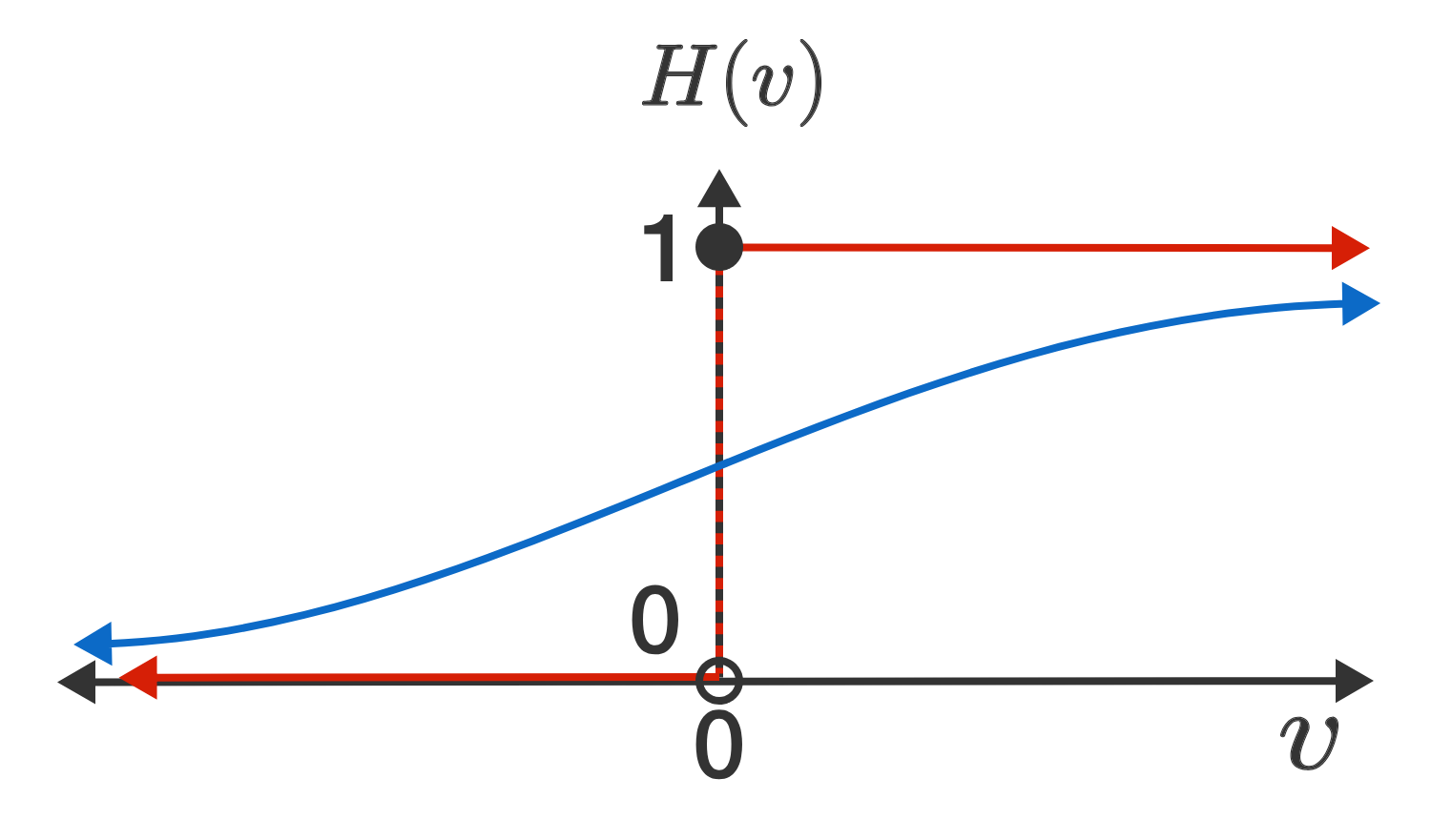# Computationally Modeling The Brain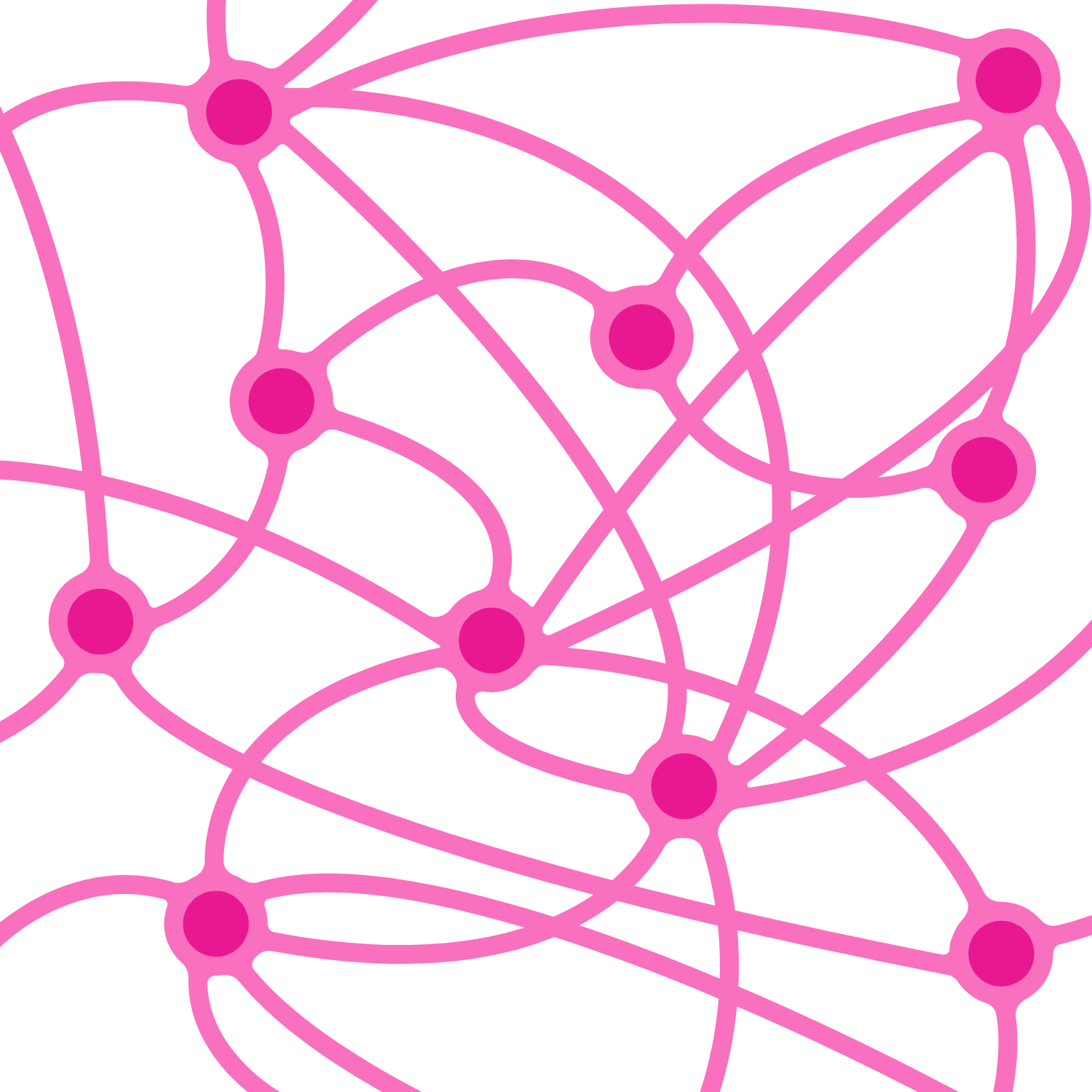Connections (or synapses) between neurons are used to pass information from the outputs of some neurons to the inputs of other neurons. Not every neuron is connected to every other neuron, and certain neurons have stronger connections to some than others.

Estimates for the number of neurons and synapses in a human brain vary widely, but there are approximately $10^{11}$ neurons in the human brain and between $10^{14}$ and $10^{15}$ synapses. Which of these values is a reasonable estimate for the average number of connections per neuron?

# Computationally Modeling The Brain

By adjusting which connections exist and how strong they are, the human brain is able to learn a huge variety of complex functions. Thus, a computational model influenced by the human brain should include simple computational units (like neurons) which are connected to one another (as with synapses). If the model can learn to adjust the strengths of those connections appropriately, it may be able to approach the power of the human brain.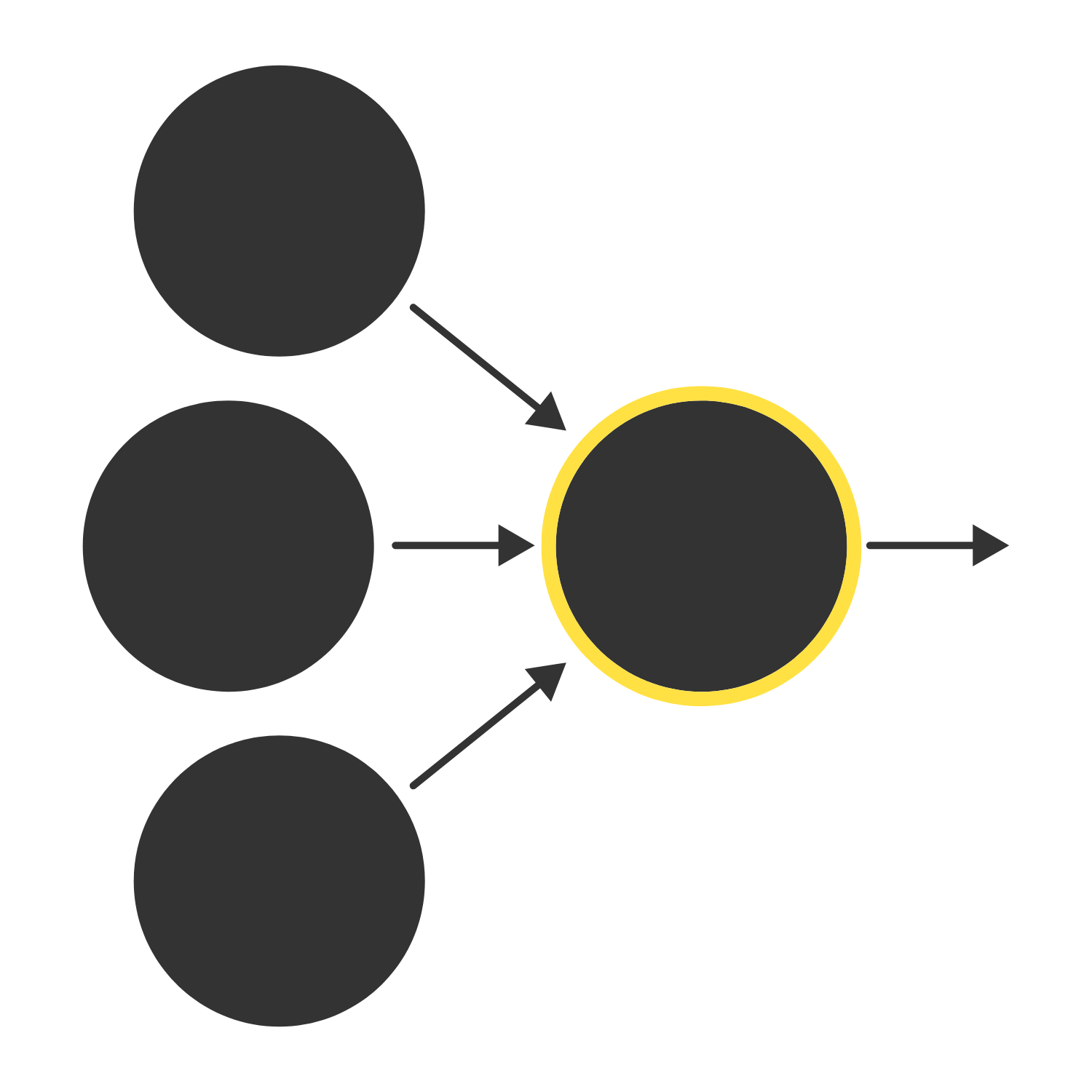In the next quiz, we’ll further develop our computational model of the neuron, nailing down how the inputs are combined via weights (representing connection strength) and finding what the possibilities are for the threshold function.

×